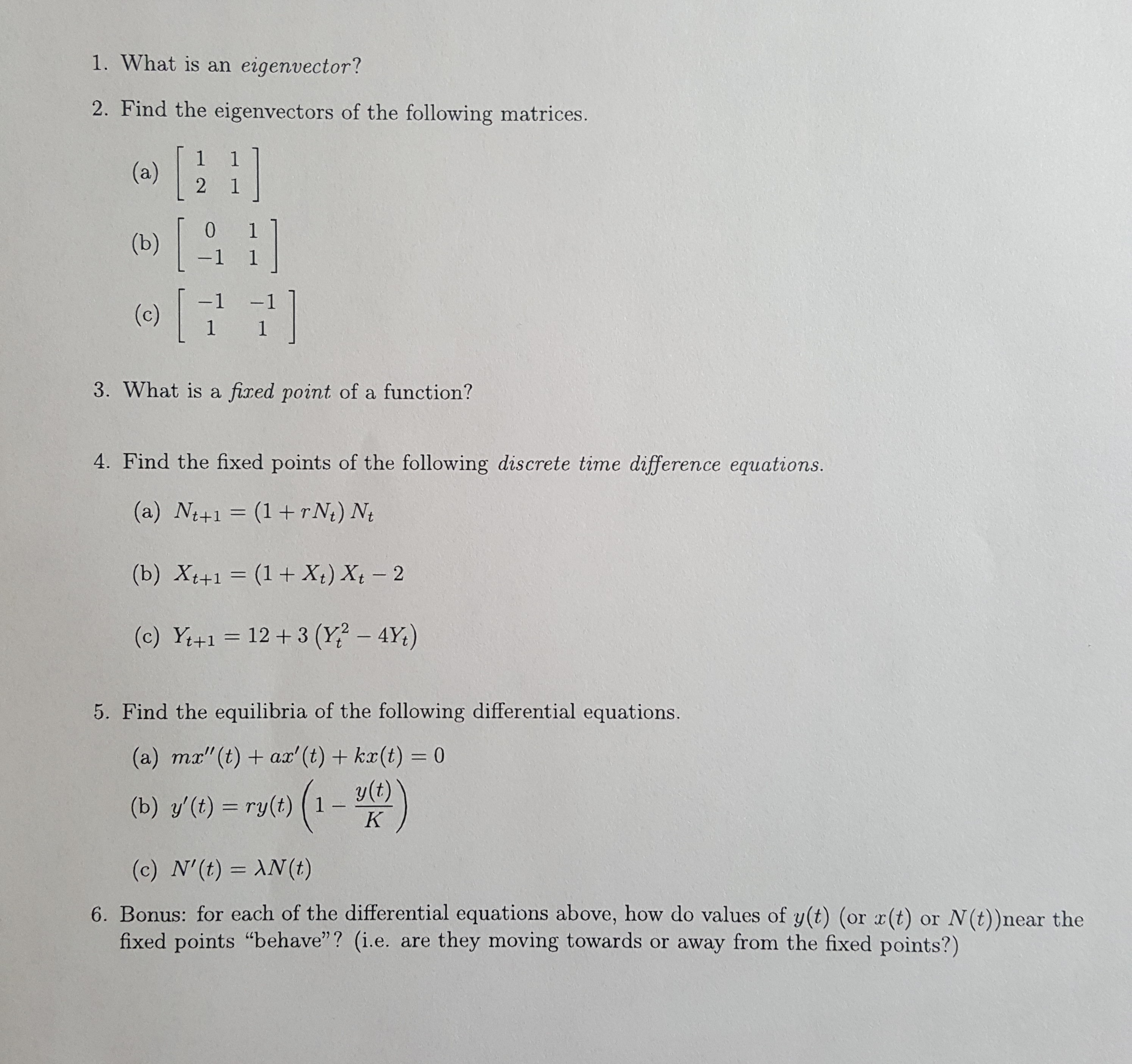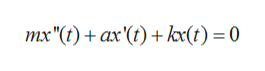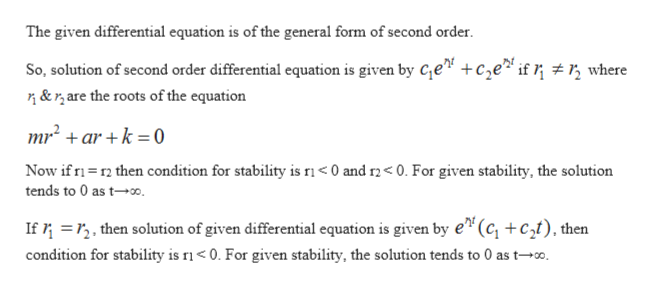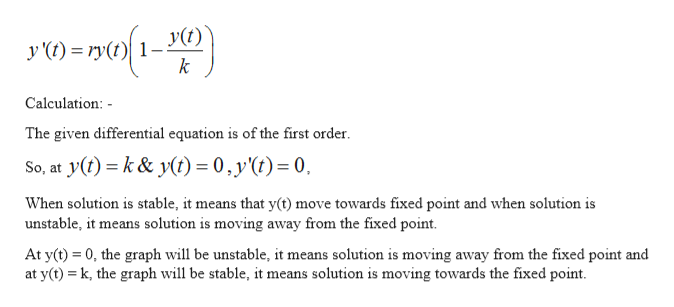1. What is an eigenvector?2. Find the eigenvectors of the following matrices.(a)21[0(b)-1 1-1(c)1 13. What is a fixed point of a function?4. Find the fixed points of the following discrete time difference equations.(a) Nt+1 (1+rN) Nt(b) Xt+1 (1 + X) Xt 212+3 (Y? - 4Y)(c) Y+15. Find the equilibria of the following differential equations.(a) mx"(t) ax' (t)+ kx(t) = 0y(t)1(b) y'(t) ry(t)K(c) N'(t) AN (t)6. Bonus: for each of the differential equations above, how do values of y(t) (or x(t)fixed points "behave"? (i.e. are they moving towards or away from the fixed points?)or N(t))near the

Question

I need help for problem 6. Thanks!help_outlineImage Transcriptionclose1. What is an eigenvector? 2. Find the eigenvectors of the following matrices. (a) 2 1 [ 0 (b) -1 1 -1 (c) 1 1 3. What is a fixed point of a function? 4. Find the fixed points of the following discrete time difference equations. (a) Nt+1 (1+rN) Nt (b) Xt+1 (1 + X) Xt 2 12+3 (Y? - 4Y) (c) Y+1 5. Find the equilibria of the following differential equations. (a) mx"(t) ax' (t)+ kx(t) = 0 y(t) 1 (b) y'(t) ry(t) K (c) N'(t) AN (t) 6. Bonus: for each of the differential equations above, how do values of y(t) (or x(t) fixed points "behave"? (i.e. are they moving towards or away from the fixed points?) or N(t))near the fullscreen
Step 1

Part(A)

Given: -help_outlineImage Transcriptioncloseтх"() + ах'(() + kx(t) %3D 0 fullscreen
Step 2

Calculation: -help_outlineImage TranscriptioncloseThe given differential equation is of the general form of second order So, solution of second order differential equation is given by Ce" +ce* if ; # r, where &r,are the roots of the equation mr2+ar +k =0 Now if r then condition for stability is ri< 0 and r2< 0. For given stability, the solution tends to 0 as t0. If2, then solution of given differential equation is given by e"(c, +c2t), then condition for stability is ri0. For given stability, the solution tends to 0 as t-. fullscreen
Step 3

Part (B)...help_outlineImage Transcriptionclosey (t) = ry(t) 1-y(t) k Calculation: The given differential equation is of the first order. So, at y() k& y(t) = 0,y(t)= 0, When solution is stable, it means that y(t) move towards fixed point and when solution is unstable, it means solution is moving away from the fixed point At y(t) 0, the graph will be unstable, it means solution is moving away from the fixed point and at y(t) k, the graph will be stable, it means solution is moving towards the fixed point fullscreen

Want to see the full answer?

See Solution

Want to see this answer and more?

Our solutions are written by experts, many with advanced degrees, and available 24/7

See Solution
Tagged in

Differential Equations